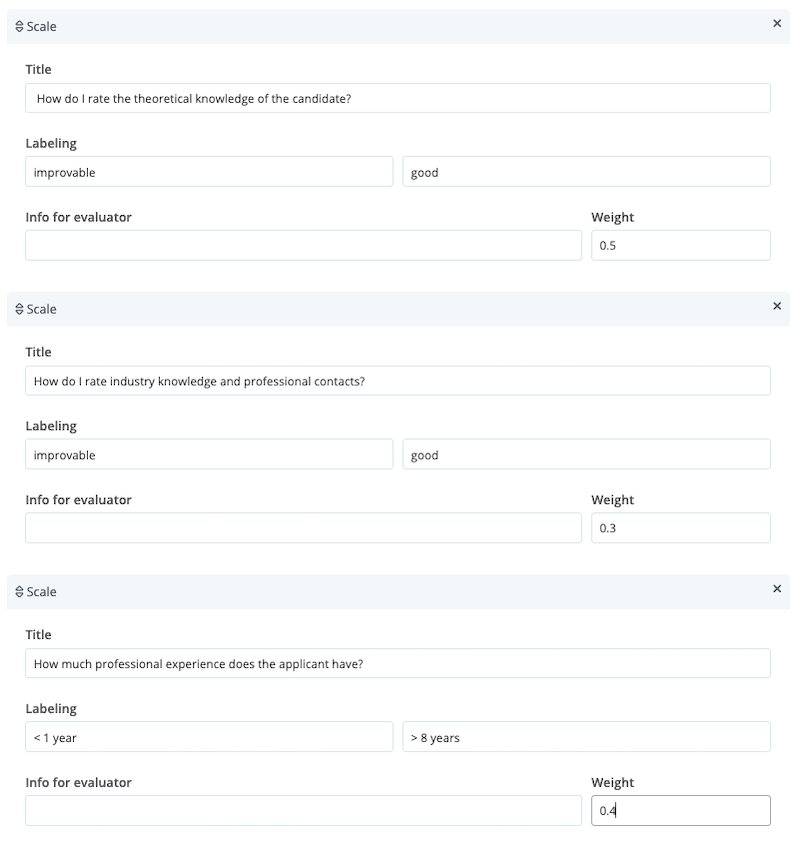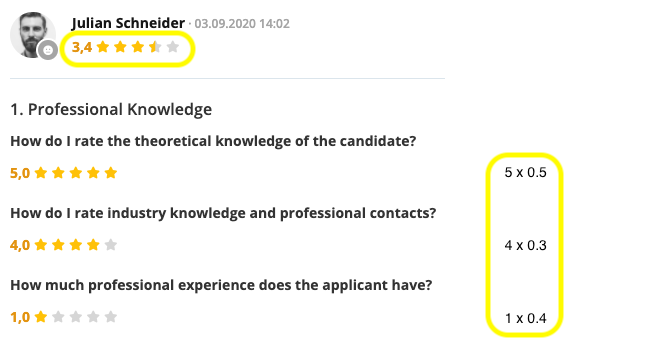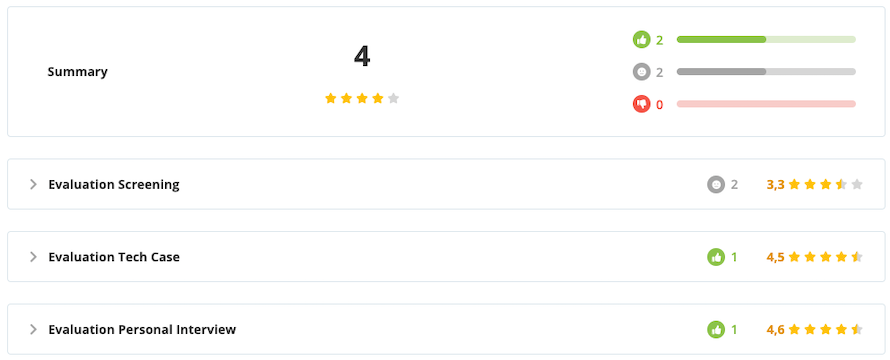# How Results in the Evaluation Forms Are Calculated

In this article, you will learn how the scales on evaluation forms are used to calculate evaluation results and how weightings contribute to this.

Personio allows you to manage feedback on candidates in an objective and structured way by using evaluation forms. Evaluation forms consist of one or more sections that you can use to evaluate a candidate in terms of user-defined criteria. A section may consist of titles, text fields and scales.

## Evaluation Form Scales

Scales are made up of a Descriptive text, labels for the lowest and highest scores and, if necessary, explanatory Info for evaluator sections. You can use weightings to determine how important each individual scale is and to what extent it should influence the evaluation as a whole.

The scales on the evaluation form for interviewers and feedback providers look like this:The descriptive text and the Info for evaluator in gray are visible straight away, while the labels for the highest and lowest scores appear when you move the mouse over them. The weighting of the scales is not visible to the evaluator.

## Defining Scale Weightings

If all your scales should have equal weighting, select a weighting of 1 for each of them.

Choose weightings between 0 and 1 if you want to assign varying levels of importance to individual scales. The result is calculated irrespective of whether the individual weightings add up to 1 or not. The example below contains several scales per section and weightings of less than 1.

Important: Please use a point for decimal values (instead of a comma).## Calculation of Scales

Calculation logic

Multiple scores within scales are combined in order to come up with the overall score for the evaluation form. The calculation logic is explained in the following example:

Three scales, for each of which a score is given:

• A (Technical competence)
• B (Professional experience)

Three weightings:

• X, Y and Z for the three scales

The result is calculated using the following formula:

[(Score Scale A x Weighting X) + (Score Scale B x Weighting Y) + (Score Scale C x Weighting Z)] / (Weighting X + Weighting Y + Weighting Z)

The results from this calculation are rounded up or down to one decimal place according to the usual rounding rules.

### Concrete examples

a. Evaluations With a Weighting of 1 EachThe following is the calculation:

[(5x1) + (4x1) + (1x1)] / (1+1+1)

= 10 / 3

= 3.33

= 3.3 when rounded

b. Evaluations With a Weighting of Less Than 1The following is the calculation:

[(5x0.5) + (4x0.3) + (1x0.4)] / (0.5+0.3+0.4)

= 4.1 / 1.2

= 3.42

= 3.4 when rounded

## Calculating Results by Evaluation Type

If multiple evaluations are completed for a candidate, a result will be calculated for each evaluation type.The following is the calculation:

[Result A (rounded) + Result B (rounded)] / Number of results

(3.0 + 3.3) / 2

= 6.3 / 2

= 3.15

= 3.2 when rounded

## Calculating the Overall Score

You will see an overall score based on all the evaluations at the top of the tab; the figure is rounded to the nearest integer. This summarizes the overall assessment of the candidate.The following is the calculation:

[Evaluation A result (rounded) + Evaluation B result (rounded) + Evaluation C result] / Number of evaluation types used

(3.3 + 3.5 + 3.2) / 3

= 10 / 3

= 3.33

= 3 when rounded